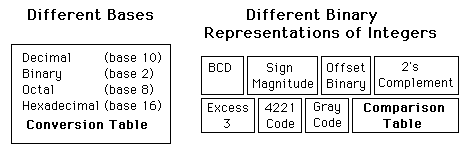# Number Systems

Digital circuits are inherently binary in nature, but several types of representations of numerical data are in use.

The representation of an unsigned integer can be done in binary, octal, decimal or hexadecimal. For display purposes, each decimal digit is often represented by a four-bit binary number in a system called binary coded decimal (BCD). Conversions between these representations can be handled in a routine manner.

The representation of signed numbers presents more problems and those problems are addressed in various ways. Some of the codes used are "sign magnitude", "offset binary", "2's complement", "excess-3", "4221", and "Gray". A table can show the display of four-bit integers.Index

Electronics concepts

Digital Circuits

 HyperPhysics*****Electricity and magnetism R Nave
Go Back

# Alphanumeric Coding

For the inherently binary world of the computer, it is necessary to put all symbols, letters, numbers, etc. into binary form. The most commonly used alphanumeric code is the ASCII code, with others like the EBCDIC code being applied in some communication applications.

 ASCII Code EBCDIC Code

 Even and Odd Parity
Index

Electronics concepts

Digital Circuits

 HyperPhysics*****Electricity and magnetism R Nave
Go Back

# Parity Checks

Errors in digital code will result in the changing of a 0 to a 1 or vice versa. One helpful method for determining if a single error of that type has ocurred is to check the evenness or oddness of the sum of the set bits. To facilitate this check an extra bit called the parity bit is added to each word in a data transmission. In the even-parity method the parity bit is chosen so that the total number of 1's including the parity bit is even. The receiver checks the parity to detect any single-bit errors. The same thing can be accomplished with an odd-parity method, so it is necessary to know which is being used in order to communicate with a host computer. It will also be necessary to know how many data bits and how many stop bits are being used.

Index

Electronics concepts

Digital Circuits

 HyperPhysics*****Electricity and magnetism R Nave
Go Back